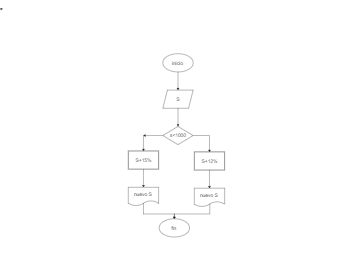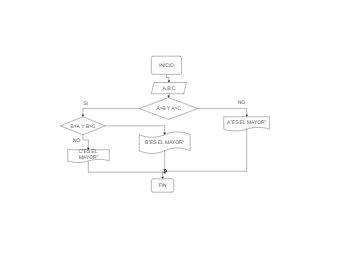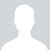## Integers Algorithm Flowchart

4
0
0
publish time: 2021-07-16Charlotte

The following algorithm chart for adding the integers from 1 to N is designed using Edraw as the free tool has predefined templates that let you create the flowchart as per the requirement. The process begins by assigning zero to a variable SUM where X is always less than N. The System will add the value of X to the current value of SUM to display the result. The System will go into the loop where X's value will be increased by +1 in every cycle until the addition is completed. Once the value of X is equal to the integer N, then the System will display SUM on the run/execute screen.

See More Related TemplatesHere is a math flowchart example. The flowchart is often used in representing algorithm, from which you could understand relative knowledge visually.
Math Flowchart Example
8
1
0Jose Luis Martinez Gonzaleztrabajo de diagrama de flujos
Dibujo1
17
0
0Sebastian Baron Lopez